ROOT   Reference GuideTSpectrum2Transform Class Reference

Advanced 2-dimensional orthogonal transform functions.

Class to carry out transforms of 2D spectra, its filtering and enhancement. It allows to calculate classic Fourier, Cosine, Sin, Hartley, Walsh, Haar transforms as well as mixed transforms (Fourier- Walsh, Fourier-Haar, Walsh-Haar, Cosine-Walsh, Cosine-Haar, Sin-Walsh and Sin-Haar). All the transforms are fast.

The algorithms in this class have been published in the following references:

1. C.V. Hampton, B. Lian, Wm. C. McHarris: Fast-Fourier-transform spectral enhancement techniques for gamma-ray spectroscopy.NIM A353 (1994) 280-284.
2. Morhac M., Matousek V., New adaptive Cosine-Walsh transform and its application to nuclear data compression, IEEE Transactions on Signal Processing 48 (2000) 2693.
3. Morhac M., Matousek V., Data compression using new fast adaptive Cosine-Haar transforms, Digital Signal Processing 8 (1998) 63.
4. Morhac M., Matousek V.: Multidimensional nuclear data compression using fast adaptive Walsh-Haar transform. Acta Physica Slovaca 51 (2001) 307.

Definition at line 16 of file TSpectrum2Transform.h.

## Public Types

enum  {
kTransformHaar =0, kTransformWalsh =1, kTransformCos =2, kTransformSin =3,
kTransformFourier =4, kTransformHartley =5, kTransformFourierWalsh =6, kTransformFourierHaar =7,
kTransformWalshHaar =8, kTransformCosWalsh =9, kTransformCosHaar =10, kTransformSinWalsh =11,
kTransformSinHaar =12, kTransformForward =0, kTransformInverse =1
}Public Types inherited from TObject
enum  {
kIsOnHeap = 0x01000000, kNotDeleted = 0x02000000, kZombie = 0x04000000, kInconsistent = 0x08000000,
kBitMask = 0x00ffffff
}

enum  { kSingleKey = BIT(0), kOverwrite = BIT(1), kWriteDelete = BIT(2) }

enum  EDeprecatedStatusBits { kObjInCanvas = BIT(3) }

enum  EStatusBits {
kCanDelete = BIT(0), kMustCleanup = BIT(3), kIsReferenced = BIT(4), kHasUUID = BIT(5),
kCannotPick = BIT(6), kNoContextMenu = BIT(8), kInvalidObject = BIT(13)
}

## Public Member Functions

TSpectrum2Transform ()
Default constructor. More...

TSpectrum2Transform (Int_t sizeX, Int_t sizeY)
The constructor creates TSpectrum2Transform object. More...

virtual ~TSpectrum2Transform ()
Destructor. More...

void Enhance (const Double_t **fSource, Double_t **fDest)
This function transforms the source spectrum. More...

void FilterZonal (const Double_t **fSource, Double_t **fDest)
This function transforms the source spectrum. More...

void SetDirection (Int_t direction)
This function sets the direction of the transform: More...

void SetEnhanceCoeff (Double_t enhanceCoeff)
This function sets the enhancement coefficient: More...

void SetFilterCoeff (Double_t filterCoeff)
This function sets the filter coefficient: More...

void SetRegion (Int_t xmin, Int_t xmax, Int_t ymin, Int_t ymax)
This function sets the filtering or enhancement region: More...

void SetTransformType (Int_t transType, Int_t degree)
This function sets the following parameters for transform: More...

void Transform (const Double_t **fSource, Double_t **fDest)
This function transforms the source spectrum. More...Public Member Functions inherited from TObject
TObject ()
TObject constructor. More...

TObject (const TObject &object)
TObject copy ctor. More...

virtual ~TObject ()
TObject destructor. More...

void AbstractMethod (const char *method) const
Use this method to implement an "abstract" method that you don't want to leave purely abstract. More...

virtual void AppendPad (Option_t *option="")
Append graphics object to current pad. More...

virtual void Browse (TBrowser *b)
Browse object. May be overridden for another default action. More...

ULong_t CheckedHash ()
Check and record whether this class has a consistent Hash/RecursiveRemove setup (*) and then return the regular Hash value for this object. More...

virtual const char * ClassName () const
Returns name of class to which the object belongs. More...

virtual void Clear (Option_t *="")

virtual TObjectClone (const char *newname="") const
Make a clone of an object using the Streamer facility. More...

virtual Int_t Compare (const TObject *obj) const
Compare abstract method. More...

virtual void Copy (TObject &object) const
Copy this to obj. More...

virtual void Delete (Option_t *option="")
Delete this object. More...

virtual Int_t DistancetoPrimitive (Int_t px, Int_t py)
Computes distance from point (px,py) to the object. More...

virtual void Draw (Option_t *option="")
Default Draw method for all objects. More...

virtual void DrawClass () const
Draw class inheritance tree of the class to which this object belongs. More...

virtual TObjectDrawClone (Option_t *option="") const
Draw a clone of this object in the current selected pad for instance with: gROOT->SetSelectedPad(gPad). More...

virtual void Dump () const
Dump contents of object on stdout. More...

virtual void Error (const char *method, const char *msgfmt,...) const
Issue error message. More...

virtual void Execute (const char *method, const char *params, Int_t *error=0)
Execute method on this object with the given parameter string, e.g. More...

virtual void Execute (TMethod *method, TObjArray *params, Int_t *error=0)
Execute method on this object with parameters stored in the TObjArray. More...

virtual void ExecuteEvent (Int_t event, Int_t px, Int_t py)
Execute action corresponding to an event at (px,py). More...

virtual void Fatal (const char *method, const char *msgfmt,...) const
Issue fatal error message. More...

virtual TObjectFindObject (const char *name) const
Must be redefined in derived classes. More...

virtual TObjectFindObject (const TObject *obj) const
Must be redefined in derived classes. More...

virtual Option_tGetDrawOption () const
Get option used by the graphics system to draw this object. More...

virtual const char * GetIconName () const
Returns mime type name of object. More...

virtual const char * GetName () const
Returns name of object. More...

virtual char * GetObjectInfo (Int_t px, Int_t py) const
Returns string containing info about the object at position (px,py). More...

virtual Option_tGetOption () const

virtual const char * GetTitle () const
Returns title of object. More...

virtual UInt_t GetUniqueID () const
Return the unique object id. More...

virtual Bool_t HandleTimer (TTimer *timer)
Execute action in response of a timer timing out. More...

virtual ULong_t Hash () const
Return hash value for this object. More...

Bool_t HasInconsistentHash () const
Return true is the type of this object is known to have an inconsistent setup for Hash and RecursiveRemove (i.e. More...

virtual void Info (const char *method, const char *msgfmt,...) const
Issue info message. More...

virtual Bool_t InheritsFrom (const char *classname) const
Returns kTRUE if object inherits from class "classname". More...

virtual Bool_t InheritsFrom (const TClass *cl) const
Returns kTRUE if object inherits from TClass cl. More...

virtual void Inspect () const
Dump contents of this object in a graphics canvas. More...

void InvertBit (UInt_t f)

virtual Bool_t IsEqual (const TObject *obj) const
Default equal comparison (objects are equal if they have the same address in memory). More...

virtual Bool_t IsFolder () const
Returns kTRUE in case object contains browsable objects (like containers or lists of other objects). More...

R__ALWAYS_INLINE Bool_t IsOnHeap () const

virtual Bool_t IsSortable () const

R__ALWAYS_INLINE Bool_t IsZombie () const

virtual void ls (Option_t *option="") const
The ls function lists the contents of a class on stdout. More...

void MayNotUse (const char *method) const
Use this method to signal that a method (defined in a base class) may not be called in a derived class (in principle against good design since a child class should not provide less functionality than its parent, however, sometimes it is necessary). More...

virtual Bool_t Notify ()
This method must be overridden to handle object notification. More...

void Obsolete (const char *method, const char *asOfVers, const char *removedFromVers) const
Use this method to declare a method obsolete. More...

void operator delete (void *ptr)
Operator delete. More...

void operator delete[] (void *ptr)
Operator delete []. More...

voidoperator new (size_t sz)

voidoperator new (size_t sz, void *vp)

voidoperator new[] (size_t sz)

voidoperator new[] (size_t sz, void *vp)

TObjectoperator= (const TObject &rhs)
TObject assignment operator. More...

virtual void Paint (Option_t *option="")
This method must be overridden if a class wants to paint itself. More...

virtual void Pop ()
Pop on object drawn in a pad to the top of the display list. More...

virtual void Print (Option_t *option="") const
This method must be overridden when a class wants to print itself. More...

virtual Int_t Read (const char *name)
Read contents of object with specified name from the current directory. More...

virtual void RecursiveRemove (TObject *obj)
Recursively remove this object from a list. More...

void ResetBit (UInt_t f)

virtual void SaveAs (const char *filename="", Option_t *option="") const
Save this object in the file specified by filename. More...

virtual void SavePrimitive (std::ostream &out, Option_t *option="")
Save a primitive as a C++ statement(s) on output stream "out". More...

void SetBit (UInt_t f)

void SetBit (UInt_t f, Bool_t set)
Set or unset the user status bits as specified in f. More...

virtual void SetDrawOption (Option_t *option="")
Set drawing option for object. More...

virtual void SetUniqueID (UInt_t uid)
Set the unique object id. More...

virtual void SysError (const char *method, const char *msgfmt,...) const
Issue system error message. More...

R__ALWAYS_INLINE Bool_t TestBit (UInt_t f) const

Int_t TestBits (UInt_t f) const

virtual void UseCurrentStyle ()
Set current style settings in this object This function is called when either TCanvas::UseCurrentStyle or TROOT::ForceStyle have been invoked. More...

virtual void Warning (const char *method, const char *msgfmt,...) const
Issue warning message. More...

virtual Int_t Write (const char *name=0, Int_t option=0, Int_t bufsize=0)
Write this object to the current directory. More...

virtual Int_t Write (const char *name=0, Int_t option=0, Int_t bufsize=0) const
Write this object to the current directory. More...

## Protected Member Functions

void BitReverse (Double_t *working_space, Int_t num)
This function carries out bit-reverse reordering of data. More...

void BitReverseHaar (Double_t *working_space, Int_t shift, Int_t num, Int_t start)
This function carries out bit-reverse reordering for Haar transform. More...

void FourCos2 (Double_t **working_matrix, Double_t *working_vector, Int_t numx, Int_t numy, Int_t direction, Int_t type)
This function calculates 2D Fourier based transforms Function parameters: More...

void Fourier (Double_t *working_space, Int_t num, Int_t hartley, Int_t direction, Int_t zt_clear)
This function calculates Fourier based transform of a part of data. More...

void General2 (Double_t **working_matrix, Double_t *working_vector, Int_t numx, Int_t numy, Int_t direction, Int_t type, Int_t degree)
This function calculates generalized (mixed) 2D transforms Function parameters: More...

Int_t GeneralExe (Double_t *working_space, Int_t zt_clear, Int_t num, Int_t degree, Int_t type)
This function calculates generalized (mixed) transforms of different degrees. More...

Int_t GeneralInv (Double_t *working_space, Int_t num, Int_t degree, Int_t type)
This function calculates inverse generalized (mixed) transforms Function parameters: More...

void Haar (Double_t *working_space, Int_t num, Int_t direction)
This function calculates Haar transform of a part of data. More...

void HaarWalsh2 (Double_t **working_matrix, Double_t *working_vector, Int_t numx, Int_t numy, Int_t direction, Int_t type)
This function calculates 2D Haar and Walsh transforms Function parameters: More...

void Walsh (Double_t *working_space, Int_t num)
This function calculates Walsh transform of a part of data. More...Protected Member Functions inherited from TObject
virtual void DoError (int level, const char *location, const char *fmt, va_list va) const
Interface to ErrorHandler (protected). More...

void MakeZombie ()

## Protected Attributes

Int_t fDegree
degree of mixed transform, applies only for Fourier-Walsh, Fourier-Haar, Walsh-Haar, Cosine-Walsh, Cosine-Haar, Sine-Walsh, Sine-Haar transforms More...

Int_t fDirection
forward or inverse transform More...

Double_t fEnhanceCoeff
multiplication coefficient applied in enhanced region; More...

Double_t fFilterCoeff
value set in the filtered region More...

Int_t fSizeX
x length of transformed data More...

Int_t fSizeY
y length of transformed data More...

Int_t fTransformType
type of transformation (Haar, Walsh, Cosine, Sine, Fourier, Hartley, Fourier-Walsh, Fourier-Haar, Walsh-Haar, Cosine-Walsh, Cosine-Haar, Sine-Walsh, Sine-Haar) More...

Int_t fXmax
last channel x of filtered or enhanced region More...

Int_t fXmin
first channel x of filtered or enhanced region More...

Int_t fYmax
last channel y of filtered or enhanced region More...

Int_t fYmin
first channel y of filtered or enhanced region More...

## Additional Inherited MembersStatic Public Member Functions inherited from TObject
static Longptr_t GetDtorOnly ()
Return destructor only flag. More...

static Bool_t GetObjectStat ()
Get status of object stat flag. More...

static void SetDtorOnly (void *obj)
Set destructor only flag. More...

static void SetObjectStat (Bool_t stat)
Turn on/off tracking of objects in the TObjectTable. More...Protected Types inherited from TObject
enum  { kOnlyPrepStep = BIT(3) }

#include <TSpectrum2Transform.h>

Inheritance diagram for TSpectrum2Transform:
[legend]

## ◆ anonymous enum

 anonymous enum
Enumerator
kTransformHaar
kTransformWalsh
kTransformCos
kTransformSin
kTransformFourier
kTransformHartley
kTransformFourierWalsh
kTransformFourierHaar
kTransformWalshHaar
kTransformCosWalsh
kTransformCosHaar
kTransformSinWalsh
kTransformSinHaar
kTransformForward
kTransformInverse

Definition at line 30 of file TSpectrum2Transform.h.

## ◆ TSpectrum2Transform() [1/2]

 TSpectrum2Transform::TSpectrum2Transform ( )

Default constructor.

Definition at line 39 of file TSpectrum2Transform.cxx.

## ◆ TSpectrum2Transform() [2/2]

 TSpectrum2Transform::TSpectrum2Transform ( Int_t sizeX, Int_t sizeY )

The constructor creates TSpectrum2Transform object.

Its sizes must be > than zero and must be power of 2. It sets default transform type to be Cosine transform. Transform parameters can be changed using setter functions.

Definition at line 57 of file TSpectrum2Transform.cxx.

## ◆ ~TSpectrum2Transform()

 TSpectrum2Transform::~TSpectrum2Transform ( )
virtual

Destructor.

Definition at line 99 of file TSpectrum2Transform.cxx.

## ◆ BitReverse()

 void TSpectrum2Transform::BitReverse ( Double_t * working_space, Int_t num )
protected

This function carries out bit-reverse reordering of data.

Function parameters:

• working_space-pointer to vector of processed data
• num-length of processed data

Definition at line 244 of file TSpectrum2Transform.cxx.

## ◆ BitReverseHaar()

 void TSpectrum2Transform::BitReverseHaar ( Double_t * working_space, Int_t shift, Int_t num, Int_t start )
protected

This function carries out bit-reverse reordering for Haar transform.

Function parameters:

• working_space-pointer to vector of processed data
• shift-shift of position of processing
• start-initial position of processed data
• num-length of processed data

Definition at line 405 of file TSpectrum2Transform.cxx.

## ◆ Enhance()

 void TSpectrum2Transform::Enhance ( const Double_t ** fSource, Double_t ** fDest )

This function transforms the source spectrum.

The calling program should fill in input parameters. Then it multiplies transformed coefficients in the given region by the given enhance_coeff and transforms it back

Function parameters:

• fSource-pointer to the matrix of source spectrum, its size should be fSizeX*fSizeY
• fDest-pointer to the matrix of destination data, its size should be fSizeX*fSizeY

### Example of enhancement

This function transforms the source spectrum (for details see Transform function). Before the Enhance function is called the class must be created by constructor and the type of the transform as well as some other parameters must be set using a set of setter functions. The Enhance function multiplies transformed coefficients in the given region (fXmin, fXmax, fYmin, fYmax) by the given fEnhancCoeff and transforms it back. Enhanced data are written into dest spectrum.

### Example - script Enhance2.c: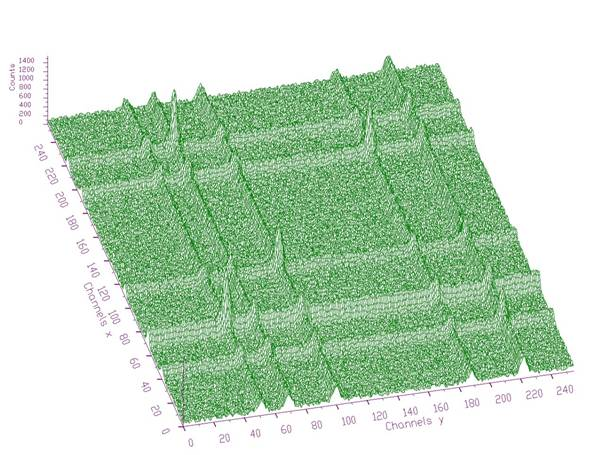Fig. 1 Original two-dimensional noisy spectrum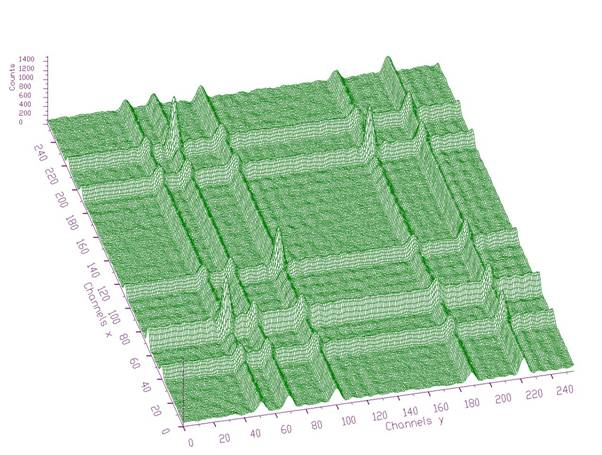Fig. 2 Enhanced spectrum of the data from Fig. 1 using Cosine transform (channels in region (0-63)x(0-63) were multiplied by 5)

#### Script:

Example to illustrate enhancement (class TSpectrumTransform2). To execute this example, do

root > .x Enhance2.C

void Enhance2() {
Int_t i, j;
Int_t nbinsx = 256;
Int_t nbinsy = 256;
Int_t xmin = 0;
Int_t xmax = nbinsx;
Int_t ymin = 0;
Int_t ymax = nbinsy;
Double_t ** source = new Double_t *[nbinsx];
Double_t ** dest = new Double_t *[nbinsx];
for (i=0;i<nbinsx;i++)
source[i]= new Double_t[nbinsy];
for (i=0;i<nbinsx;i++)
dest[i]= new Double_t[nbinsy];
TH2F *trans = new TH2F("trans","Background estimation",nbinsx,xmin,xmax,nbinsy,ymin,ymax);
TFile *f = new TFile("TSpectrum2.root");
trans=(TH2F*)f->Get("back3;1");
TCanvas *Tr = new TCanvas("Transform","Illustration of transform function",10,10,1000,700);
for (i = 0; i < nbinsx; i++){
for (j = 0; j < nbinsy; j++){
source[i][j] = trans->GetBinContent(i + 1,j + 1);
}
}
TSpectrumTransform2 *t = new TSpectrumTransform2(256,256);
t->SetTransformType(t->kTransformCos,0);
t->SetRegion(0,63,0,63);
t->SetEnhanceCoeff(5);
t->Enhance(source,dest);
trans->Draw("SURF");
}

Definition at line 2445 of file TSpectrum2Transform.cxx.

## ◆ FilterZonal()

 void TSpectrum2Transform::FilterZonal ( const Double_t ** fSource, Double_t ** fDest )

This function transforms the source spectrum.

The calling program should fill in input parameters. Then it sets transformed coefficients in the given region to the given filter_coeff and transforms it back Filtered data are written into dest spectrum.

Function parameters:

• fSource-pointer to the matrix of source spectrum, its size should be fSizeX*fSizeY
• fDest-pointer to the matrix of destination data, its size should be fSizeX*fSizeY

### Example of zonal filtering

This function transforms the source spectrum (for details see Transform function). Before the FilterZonal function is called the class must be created by constructor and the type of the transform as well as some other parameters must be set using a set of setter functions. The FilterZonal function sets transformed coefficients in the given region (fXmin, fXmax) to the given fFilterCoeff and transforms it back. Filtered data are written into dest spectrum.

### Example - script Fitler2.c:Fig. 1 Original two-dimensional noisy spectrum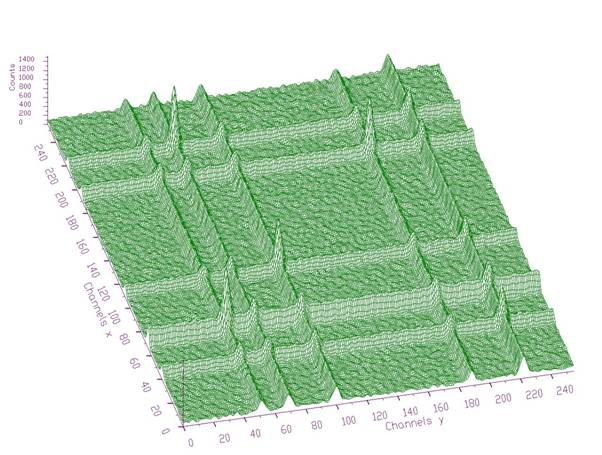Fig. 2 Filtered spectrum using Cosine transform and zonal filtration (channels in regions (128-255)x(0-255) and (0-255)x(128-255) were set to 0).

#### Script:

Example to illustrate zonal filtration (class TSpectrumTransform2). To execute this example, do

root > .x Filter2.C

void Filter2() {
Int_t i, j;
Int_t nbinsx = 256;
Int_t nbinsy = 256;
Int_t xmin = 0;
Int_t xmax = nbinsx;
Int_t ymin = 0;
Int_t ymax = nbinsy;
Double_t ** source = new Double_t *[nbinsx];
Double_t ** dest = new Double_t *[nbinsx];
for (i=0;i<nbinsx;i++)
source[i] = new Double_t[nbinsy];
for (i=0;i<nbinsx;i++)
dest[i]= new Double_t[nbinsy];
TH2F *trans = new TH2F("trans","Background estimation",nbinsx,xmin,xmax,nbinsy,ymin,ymax);
TFile *f = new TFile("TSpectrum2.root");
trans=(TH2F*)f->Get("back3;1");
TCanvas *Tr = new TCanvas("Transform","Illustration of transform function",10,10,1000,700);
for (i = 0; i < nbinsx;i++){
for (j = 0; j <nbinsy; j++){
source[i][j] = trans->GetBinContent(i + 1,j+ 1);
}
}
TSpectrumTransform2 *t = new TSpectrumTransform2(256,256);
t->SetTransformType(t->kTransformCos,0);
t->SetRegion(0,255,128,255);
t->FilterZonal(source,dest);
for (i = 0; i < nbinsx; i++){
for (j = 0; j <nbinsy; j++){
source[i][j] = dest[i][j];
}
}
t->SetRegion(128,255,0,255);
t->FilterZonal(source,dest);
trans->Draw("SURF");
}

Definition at line 2114 of file TSpectrum2Transform.cxx.

## ◆ FourCos2()

 void TSpectrum2Transform::FourCos2 ( Double_t ** working_matrix, Double_t * working_vector, Int_t numx, Int_t numy, Int_t direction, Int_t type )
protected

This function calculates 2D Fourier based transforms Function parameters:

• working_matrix-pointer to matrix of transformed data
• working_vector-pointer to vector where the data are processed
• numx,numy-lengths of processed data
• direction-forward or inverse
• type-type of transform (see manual)

Definition at line 778 of file TSpectrum2Transform.cxx.

## ◆ Fourier()

 void TSpectrum2Transform::Fourier ( Double_t * working_space, Int_t num, Int_t hartley, Int_t direction, Int_t zt_clear )
protected

This function calculates Fourier based transform of a part of data.

Function parameters:

• working_space-pointer to vector of transformed data
• num-length of processed data
• hartley-1 if it is Hartley transform, 0 otherwise
• direction-forward or inverse transform

Definition at line 283 of file TSpectrum2Transform.cxx.

## ◆ General2()

 void TSpectrum2Transform::General2 ( Double_t ** working_matrix, Double_t * working_vector, Int_t numx, Int_t numy, Int_t direction, Int_t type, Int_t degree )
protected

This function calculates generalized (mixed) 2D transforms Function parameters:

• working_matrix-pointer to matrix of transformed data
• working_vector-pointer to vector where the data are processed
• numx,numy-lengths of processed data
• direction-forward or inverse
• type-type of transform (see manual)
• degree-degree of transform (see manual)

Definition at line 1046 of file TSpectrum2Transform.cxx.

## ◆ GeneralExe()

 Int_t TSpectrum2Transform::GeneralExe ( Double_t * working_space, Int_t zt_clear, Int_t num, Int_t degree, Int_t type )
protected

This function calculates generalized (mixed) transforms of different degrees.

Function parameters:

• working_space-pointer to vector of transformed data
• zt_clear-flag to clear imaginary data before staring
• num-length of processed data
• degree-degree of transform (see manual)
• type-type of mixed transform (see manual)

Definition at line 451 of file TSpectrum2Transform.cxx.

## ◆ GeneralInv()

 Int_t TSpectrum2Transform::GeneralInv ( Double_t * working_space, Int_t num, Int_t degree, Int_t type )
protected

This function calculates inverse generalized (mixed) transforms Function parameters:

• working_space-pointer to vector of transformed data
• num-length of processed data
• degree-degree of transform (see manual)
• type-type of mixed transform (see manual)

Definition at line 568 of file TSpectrum2Transform.cxx.

## ◆ Haar()

 void TSpectrum2Transform::Haar ( Double_t * working_space, Int_t num, Int_t direction )
protected

This function calculates Haar transform of a part of data.

Function parameters:

• working_space-pointer to vector of transformed data
• num-length of processed data
• direction-forward or inverse transform

Definition at line 111 of file TSpectrum2Transform.cxx.

## ◆ HaarWalsh2()

 void TSpectrum2Transform::HaarWalsh2 ( Double_t ** working_matrix, Double_t * working_vector, Int_t numx, Int_t numy, Int_t direction, Int_t type )
protected

This function calculates 2D Haar and Walsh transforms Function parameters:

• working_matrix-pointer to matrix of transformed data
• working_vector-pointer to vector where the data are processed
• numx,numy-lengths of processed data
• direction-forward or inverse
• type-type of transform (see manual)

Definition at line 687 of file TSpectrum2Transform.cxx.

## ◆ SetDirection()

 void TSpectrum2Transform::SetDirection ( Int_t direction )

This function sets the direction of the transform:

• direction (forward or inverse)

Definition at line 2768 of file TSpectrum2Transform.cxx.

## ◆ SetEnhanceCoeff()

 void TSpectrum2Transform::SetEnhanceCoeff ( Double_t enhanceCoeff )

This function sets the enhancement coefficient:

• enhanceCoeff - after the transform the enhanced region (xmin, xmax, ymin, ymax) is multiplied by this coefficient. Applies only for enhancement operation.

Definition at line 2790 of file TSpectrum2Transform.cxx.

## ◆ SetFilterCoeff()

 void TSpectrum2Transform::SetFilterCoeff ( Double_t filterCoeff )

This function sets the filter coefficient:

• filterCoeff - after the transform the filtered region (xmin, xmax, ymin, ymax) is replaced by this coefficient. Applies only for filtereng operation.

Definition at line 2781 of file TSpectrum2Transform.cxx.

## ◆ SetRegion()

 void TSpectrum2Transform::SetRegion ( Int_t xmin, Int_t xmax, Int_t ymin, Int_t ymax )

This function sets the filtering or enhancement region:

• xmin, xmax, ymin, ymax

Definition at line 2748 of file TSpectrum2Transform.cxx.

## ◆ SetTransformType()

 void TSpectrum2Transform::SetTransformType ( Int_t transType, Int_t degree )

This function sets the following parameters for transform:

• transType - type of transform (Haar, Walsh, Cosine, Sine, Fourier, Hartley, Fourier-Walsh, Fourier-Haar, Walsh-Haar, Cosine-Walsh, Cosine-Haar, Sine-Walsh, Sine-Haar)
• degree - degree of mixed transform, applies only for Fourier-Walsh, Fourier-Haar, Walsh-Haar, Cosine-Walsh, Cosine-Haar, Sine-Walsh, Sine-Haar transforms

Definition at line 2715 of file TSpectrum2Transform.cxx.

## ◆ Transform()

 void TSpectrum2Transform::Transform ( const Double_t ** fSource, Double_t ** fDest )

This function transforms the source spectrum.

The calling program should fill in input parameters. Transformed data are written into dest spectrum.

Function parameters:

• fSource-pointer to the matrix of source spectrum, its size should be fSizex*fSizey except for inverse FOURIER, FOUR-WALSH, FOUR-HAAR transform. These need fSizex*2*fSizey length to supply real and imaginary coefficients.
• fDest-pointer to the matrix of destination data, its size should be fSizex*fSizey except for direct FOURIER, FOUR-WALSh, FOUR-HAAR. These need fSizex*2*fSizey length to store real and imaginary coefficients
• fSizex,fSizey-basic dimensions of source and dest spectra

### Transform methods

Goal: to analyse experimental data using orthogonal transforms

• orthogonal transforms can be successfully used for the processing of nuclear spectra (not only)
• they can be used to remove high frequency noise, to increase signal-to-background ratio as well as to enhance low intensity components , to carry out e.g. Fourier analysis etc.
• we have implemented the function for the calculation of the commonly used orthogonal transforms as well as functions for the filtration and enhancement of experimental data

#### References:

 C.V. Hampton, B. Lian, Wm. C. McHarris: Fast-Fourier-transform spectral enhancement techniques for gamma-ray spectroscopy. NIM A353 (1994) 280-284.

 Morhac M., Matouoek V., New adaptive Cosine-Walsh transform and its application to nuclear data compression, IEEE Transactions on Signal Processing 48 (2000) 2693.

 Morhac M., Matouoek V., Data compression using new fast adaptive Cosine-Haar transforms, Digital Signal Processing 8 (1998) 63.

 Morhac M., Matouoek V.: Multidimensional nuclear data compression using fast adaptive Walsh-Haar transform. Acta Physica Slovaca 51 (2001) 307.

### Example 1 - script Transform2.c: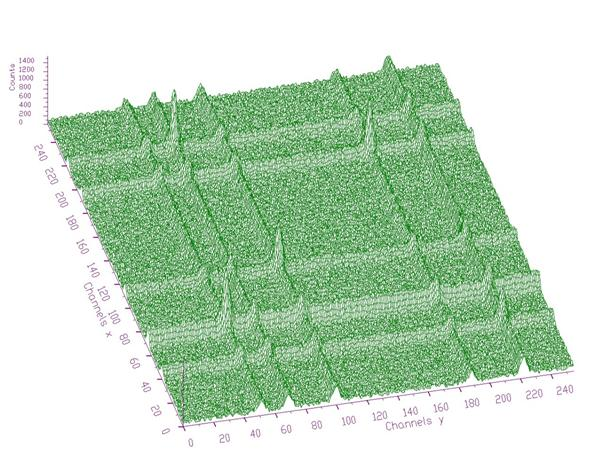Fig. 1 Original two-dimensional noisy spectrum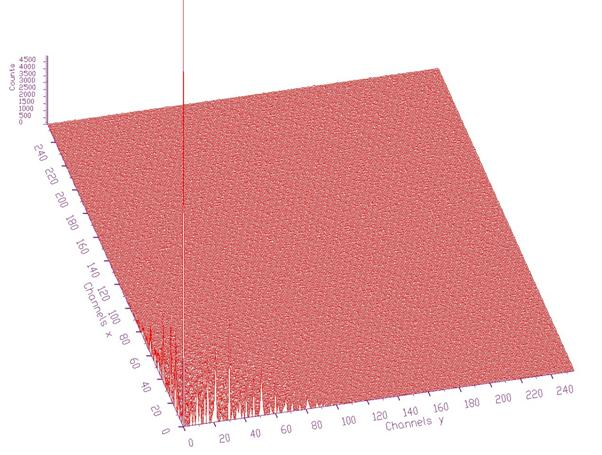Fig. 2 Transformed spectrum from Fig. 1 using Cosine transform. Energy of the transformed data is concentrated around the beginning of the coordinate system

#### Script:

Example to illustrate Transform function (class TSpectrumTransform2). To execute this example, do

root > .x Transform2.C

void Transform2() {
Int_t i, j;
Int_t nbinsx = 256;
Int_t nbinsy = 256;
Int_t xmin = 0;
Int_t xmax = nbinsx;
Int_t ymin = 0;
Int_t ymax = nbinsy;
Double_t ** source = new Double_t *[nbinsx];
Double_t ** dest = new Double_t *[nbinsx];
for (i=0;i<nbinsx;i++)
source[i]=newDouble_t[nbinsy];
for (i=0;i<nbinsx;i++)
dest[i]=newDouble_t[nbinsy];
TH2F *trans = newTH2F("trans","Background estimation",nbinsx,xmin,xmax,nbinsy,ymin,ymax);
TFile *f = new TFile("TSpectrum2.root");
trans=(TH2F*)f->Get("back3;1");
TCanvas *Tr = new TCanvas("Transform","Illustration of transform function",10,10,1000,700);
for (i = 0; i < nbinsx; i++){
for (j = 0; j < nbinsy; j++){
source[i][j] = trans->GetBinContent(i + 1,j + 1);
}
}
TSpectrumTransform2 *t = new TSpectrumTransform2(256,256);
t->SetTransformType(t->kTransformCos,0);
t->SetDirection(t->kTransformForward);
t->Transform(source,dest);
for (i = 0; i < nbinsx; i++){
for (j = 0; j < nbinsy; j++){
trans->SetBinContent(i+ 1, j + 1,dest[i][j]);
}
}
trans->Draw("SURF");
}

Definition at line 1754 of file TSpectrum2Transform.cxx.

## ◆ Walsh()

 void TSpectrum2Transform::Walsh ( Double_t * working_space, Int_t num )
protected

This function calculates Walsh transform of a part of data.

Function parameters:

• working_space-pointer to vector of transformed data
• num-length of processed data

Definition at line 190 of file TSpectrum2Transform.cxx.

## ◆ fDegree

 Int_t TSpectrum2Transform::fDegree
protected

degree of mixed transform, applies only for Fourier-Walsh, Fourier-Haar, Walsh-Haar, Cosine-Walsh, Cosine-Haar, Sine-Walsh, Sine-Haar transforms

Definition at line 21 of file TSpectrum2Transform.h.

## ◆ fDirection

 Int_t TSpectrum2Transform::fDirection
protected

forward or inverse transform

Definition at line 22 of file TSpectrum2Transform.h.

## ◆ fEnhanceCoeff

 Double_t TSpectrum2Transform::fEnhanceCoeff
protected

multiplication coefficient applied in enhanced region;

Definition at line 28 of file TSpectrum2Transform.h.

## ◆ fFilterCoeff

 Double_t TSpectrum2Transform::fFilterCoeff
protected

value set in the filtered region

Definition at line 27 of file TSpectrum2Transform.h.

## ◆ fSizeX

 Int_t TSpectrum2Transform::fSizeX
protected

x length of transformed data

Definition at line 18 of file TSpectrum2Transform.h.

## ◆ fSizeY

 Int_t TSpectrum2Transform::fSizeY
protected

y length of transformed data

Definition at line 19 of file TSpectrum2Transform.h.

## ◆ fTransformType

 Int_t TSpectrum2Transform::fTransformType
protected

type of transformation (Haar, Walsh, Cosine, Sine, Fourier, Hartley, Fourier-Walsh, Fourier-Haar, Walsh-Haar, Cosine-Walsh, Cosine-Haar, Sine-Walsh, Sine-Haar)

Definition at line 20 of file TSpectrum2Transform.h.

## ◆ fXmax

 Int_t TSpectrum2Transform::fXmax
protected

last channel x of filtered or enhanced region

Definition at line 24 of file TSpectrum2Transform.h.

## ◆ fXmin

 Int_t TSpectrum2Transform::fXmin
protected

first channel x of filtered or enhanced region

Definition at line 23 of file TSpectrum2Transform.h.

## ◆ fYmax

 Int_t TSpectrum2Transform::fYmax
protected

last channel y of filtered or enhanced region

Definition at line 26 of file TSpectrum2Transform.h.

## ◆ fYmin

 Int_t TSpectrum2Transform::fYmin
protected

first channel y of filtered or enhanced region

Definition at line 25 of file TSpectrum2Transform.h.

Libraries for TSpectrum2Transform:[legend]

The documentation for this class was generated from the following files:
ymax
float ymax
Definition: THbookFile.cxx:95
f
#define f(i)
Definition: RSha256.hxx:104
dest
#define dest(otri, vertexptr)
Definition: triangle.c:1040
TH2::GetBinContent
virtual Double_t GetBinContent(Int_t bin) const
Return content of bin number bin.
Definition: TH2.h:88
TH2F
2-D histogram with a float per channel (see TH1 documentation)}
Definition: TH2.h:251
xmax
float xmax
Definition: THbookFile.cxx:95
Int_t
int Int_t
Definition: RtypesCore.h:45
xmin
float xmin
Definition: THbookFile.cxx:95
TFile
A ROOT file is a suite of consecutive data records (TKey instances) with a well defined format.
Definition: TFile.h:54
ymin
float ymin
Definition: THbookFile.cxx:95
Double_t
double Double_t
Definition: RtypesCore.h:59
TCanvas
The Canvas class.
Definition: TCanvas.h:23
TH2::SetBinContent
virtual void SetBinContent(Int_t bin, Double_t content)
Set bin content.
Definition: TH2.cxx:2507
TH1::Draw
virtual void Draw(Option_t *option="")
Draw this histogram with options.
Definition: TH1.cxx:3073